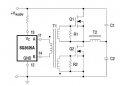# Gate drive transformer secondary parameters

#### picstudent

Joined Feb 3, 2009
91I am doing something like this T2 is not fully shown. My question is about T1
Especially the secondary. transformer ratio is 1:1.
How we can make sure the transformer secondary is capable of supplying the gate drive requirements of the MOSFET.
There should be relation between frequency, gate charge requirement, ( secondary current) etc.
What is the design procedure here?.

#### schmitt trigger

Joined Jul 12, 2010
510
One of the most important aspects, to prevent saturation, is the volt-time product, usually specified in volts-microseconds.

Essentially is the maximum "on" time multiplied by the maximum primary voltage.

A simple example: You are switching at 50 Khz, or a period of 20 microseconds.
If your maximum duty cycle is 50%, then your on time will be 10 microseconds.

If the primary applied voltage is 12 volts, then you are applying 120 volt-microseconds.

You require a transformer with a rating higher than that, with some margin. For the example above you would choose a transformer rated for 150 volts-microseconds.

•picstudent

#### picstudent

Joined Feb 3, 2009
91
One of the most important aspects, to prevent saturation, is the volt-time product, usually specified in volts-microseconds.

Essentially is the maximum "on" time multiplied by the maximum primary voltage.

A simple example: You are switching at 50 Khz, or a period of 20 microseconds.
If your maximum duty cycle is 50%, then your on time will be 10 microseconds.

If the primary applied voltage is 12 volts, then you are applying 120 volt-microseconds.

You require a transformer with a rating higher than that, with some margin. For the example above you would choose a transformer rated for 150 volts-microseconds.
That is taken care of. My frequency is 25 kHz. So avoiding saturation I got 20 Turns in my torroid. So since Ratio is 1:1, secondary also will have to have same number of turns.
But my main doubt is how we make sure or "calculate" the secondary can supply the Gate drive requirement of the MOSFETs/